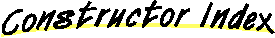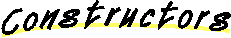# Class IntSet

```java.lang.Object
|
+----IntSet
```

public class IntSet
extends Object
implements Cloneable
A set of non-negative (small) int's. The set automatically grows. Based on java.util.BitSet.

##IntSet()
Creates an empty set.IntSet(int)
Initialize with 1 number in the setIntSet(int, int)
Initialize with 2 numbers in the setIntSet(int, int, int)
Initialize with 3 numbers in the setIntSet(int, int, int, int)
Initialize with 4 numbers in the setIntSet(int, int, int, int, int)
Initialize with 5 numbers in the setIntSet(int, int, int, int, int, int)
Initialize with 6 numbers in the setIntSet(int, int, int, int, int, int, int)
Initialize with 7 numbers in the setIntSet(int, int, int, int, int, int, int, int)
Initialize with 8 numbers in the setIntSet(int, int, int, int, int, int, int, int, int)
Initialize with 9 numbers in the setIntSet(int, int, int, int, int, int, int, int, int, int)
Initialize with 10 numbers in the setIntSet(int, int, int, int, int, int, int, int, int, int, int)
Initialize with 11 numbers in the setIntSet(int, int, int, int, int, int, int, int, int, int, int, int)
Initialize with 12 numbers in the setIntSet(int[])
Creates a set initialized from an array of integers.

##add(int)add(IntSet)
Add a set to the current set.clone()
Clones the IntSet.delete(int)
Removes a numberdelete(IntSet)
Delete all members of a set from the current set.equals(Object)
Compares this object against the specified object.hashCode()
Gets the hashcode.hasMember(int)
Checks for presence of a numberrestrictTo(IntSet)
Restrict the set to the intersection with another setsize()
Calculates and returns the set's sizetoString()
Converts the IntSet to a String.

##IntSet
``` public IntSet()
```
Creates an empty set.IntSet
``` public IntSet(int x[])
```
Creates a set initialized from an array of integers.

Parameters:
x - the array of integers to intialize fromIntSet
``` public IntSet(int n0,
int n1,
int n2,
int n3,
int n4,
int n5,
int n6,
int n7,
int n8,
int n9,
int n10,
int n11)
```
Initialize with 12 numbers in the setIntSet
``` public IntSet(int n0,
int n1,
int n2,
int n3,
int n4,
int n5,
int n6,
int n7,
int n8,
int n9,
int n10)
```
Initialize with 11 numbers in the setIntSet
``` public IntSet(int n0,
int n1,
int n2,
int n3,
int n4,
int n5,
int n6,
int n7,
int n8,
int n9)
```
Initialize with 10 numbers in the setIntSet
``` public IntSet(int n0,
int n1,
int n2,
int n3,
int n4,
int n5,
int n6,
int n7,
int n8)
```
Initialize with 9 numbers in the setIntSet
``` public IntSet(int n0,
int n1,
int n2,
int n3,
int n4,
int n5,
int n6,
int n7)
```
Initialize with 8 numbers in the setIntSet
``` public IntSet(int n0,
int n1,
int n2,
int n3,
int n4,
int n5,
int n6)
```
Initialize with 7 numbers in the setIntSet
``` public IntSet(int n0,
int n1,
int n2,
int n3,
int n4,
int n5)
```
Initialize with 6 numbers in the setIntSet
``` public IntSet(int n0,
int n1,
int n2,
int n3,
int n4)
```
Initialize with 5 numbers in the setIntSet
``` public IntSet(int n0,
int n1,
int n2,
int n3)
```
Initialize with 4 numbers in the setIntSet
``` public IntSet(int n0,
int n1,
int n2)
```
Initialize with 3 numbers in the setIntSet
``` public IntSet(int n0,
int n1)
```
Initialize with 2 numbers in the setIntSet
``` public IntSet(int n0)
```
Initialize with 1 number in the set

##add
``` public IntSet add(int x)
```

Parameters:
x - the number to adddelete
``` public IntSet delete(int x)
```
Removes a number

Parameters:
x - the number to removehasMember
``` public boolean hasMember(int x)
```
Checks for presence of a number

Parameters:
x - the number to checkrestrictTo
``` public IntSet restrictTo(IntSet set)
```
Restrict the set to the intersection with another set

Parameters:
set - the set to intersect withadd
``` public IntSet add(IntSet set)
```
Add a set to the current set.

Parameters:
set - the set to be addeddelete
``` public IntSet delete(IntSet set)
```
Delete all members of a set from the current set.

Parameters:
set - the set to be removedhashCode
``` public int hashCode()
```
Gets the hashcode.

Overrides:
hashCode in class Objectsize
``` public int size()
```
Calculates and returns the set's sizeequals
``` public boolean equals(Object obj)
```
Compares this object against the specified object.

Parameters:
obj - the object to commpare with
Returns:
true if the objects are the same; false otherwise.
Overrides:
equals in class Objectclone
``` public Object clone()
```
Clones the IntSet.

Overrides:
clone in class ObjecttoString
``` public String toString()
```
Converts the IntSet to a String.

Overrides:
toString in class Object